Lines parallel x or y axis

Chapter 4 Class 9 Linear Equations in Two Variables
Concept wise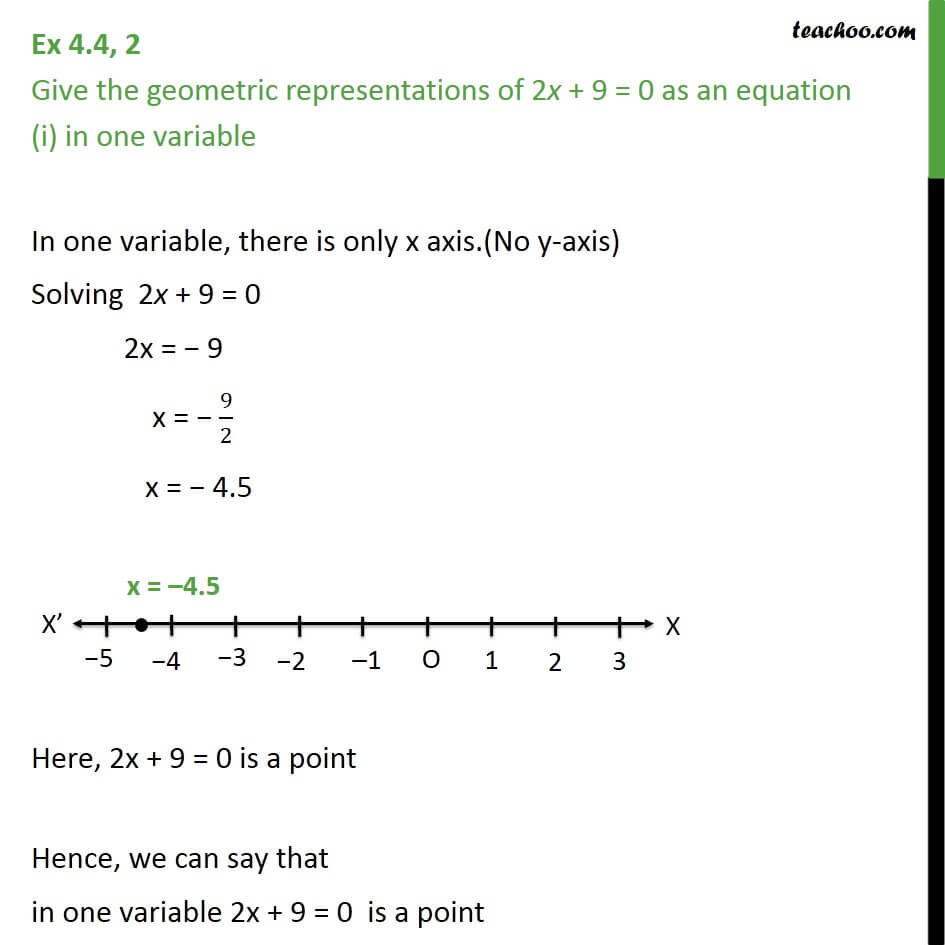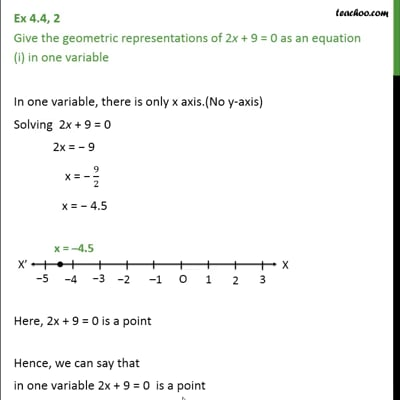This video is only available for Teachoo black users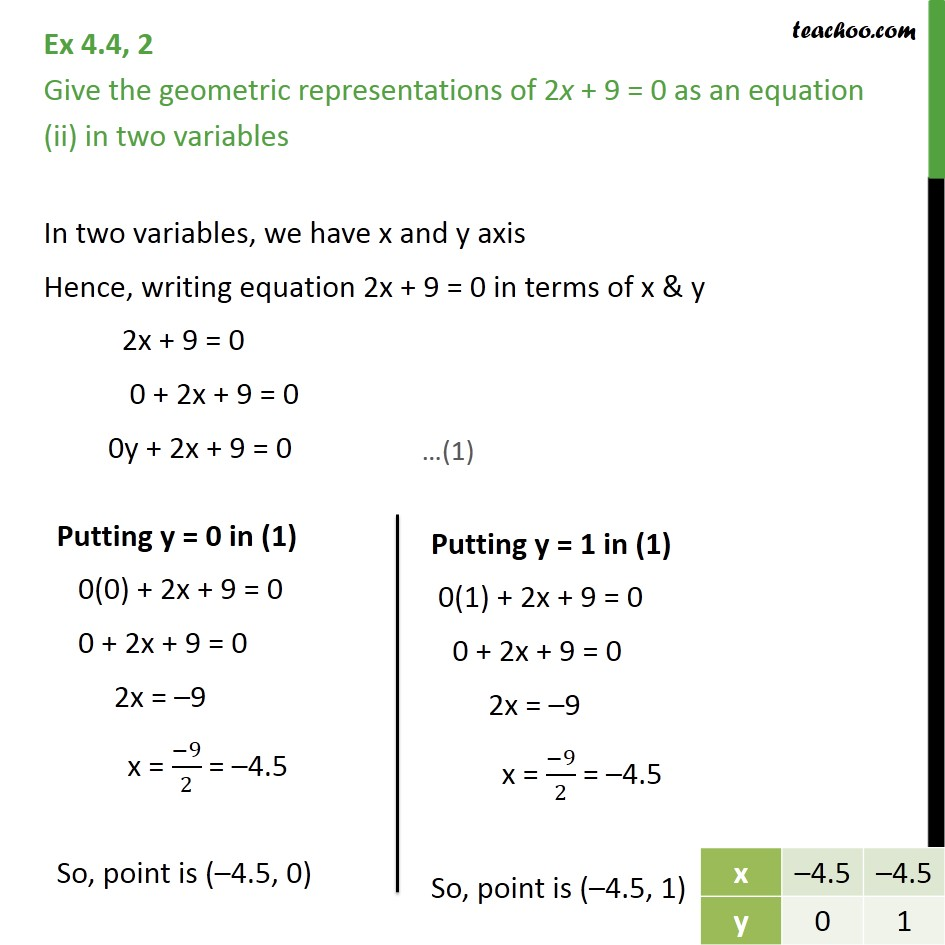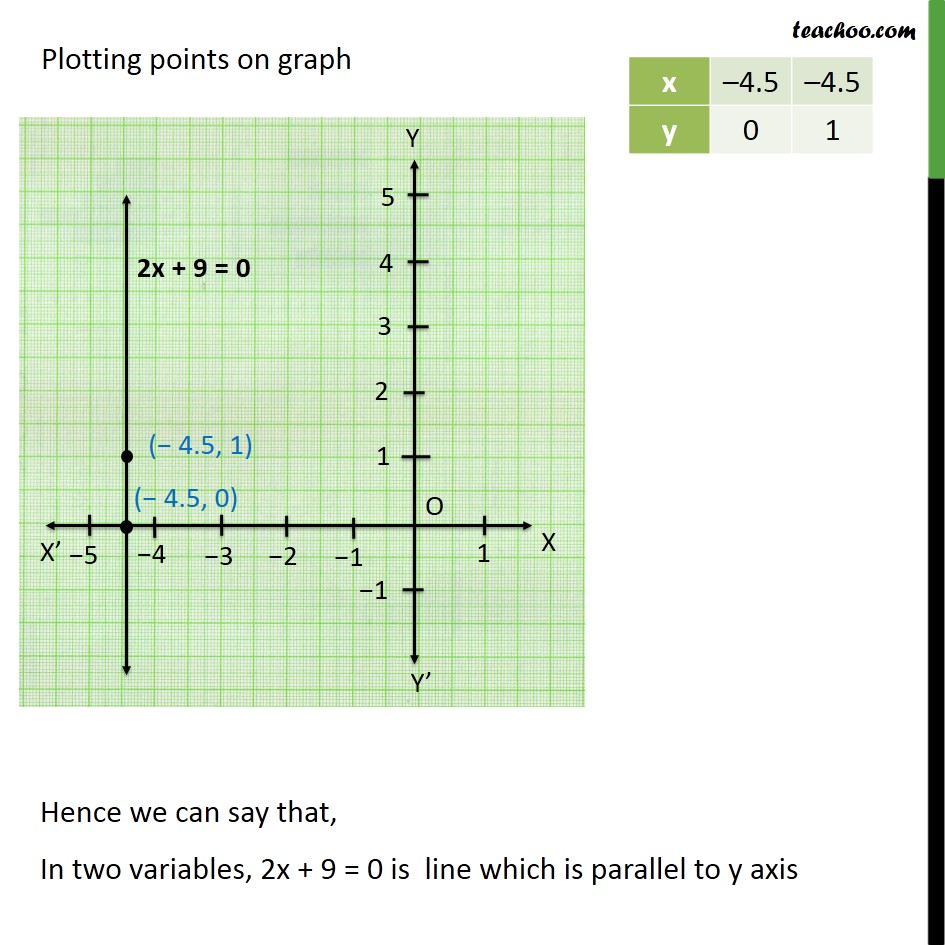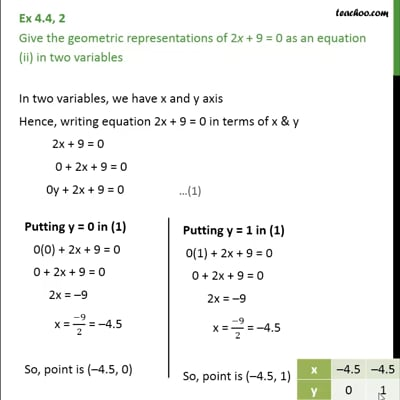This video is only available for Teachoo black users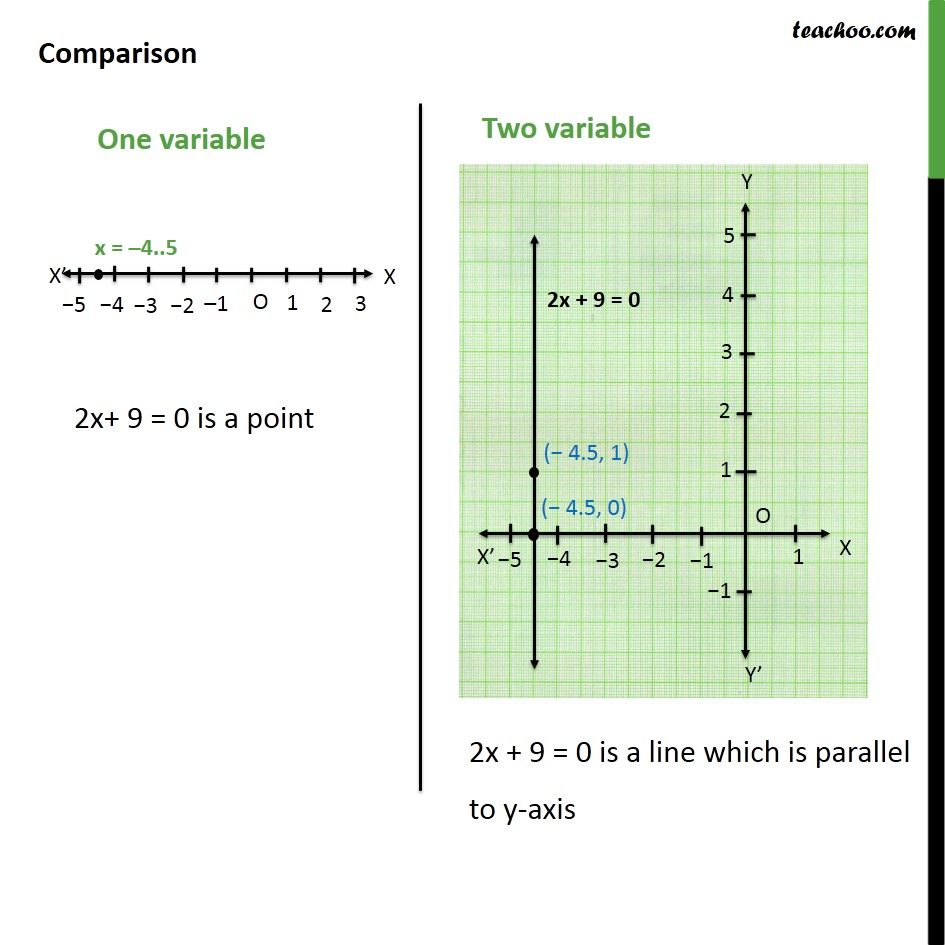This video is only available for Teachoo black users

Introducing your new favourite teacher - Teachoo Black, at only ₹83 per month

### Transcript

Ex 4.4, 2 Give the geometric representations of 2x + 9 = 0 as an equation (i) in one variable In one variable, there is only x axis.(No y-axis) Solving 2x + 9 = 0 2x = 9 x = 9/2 x = 4.5 Here, 2x + 9 = 0 is a point Hence, we can say that in one variable 2x + 9 = 0 is a point Ex 4.4, 2 Give the geometric representations of 2x + 9 = 0 as an equation (ii) in two variables In two variables, we have x and y axis Hence, writing equation 2x + 9 = 0 in terms of x & y 2x + 9 = 0 0 + 2x + 9 = 0 0y + 2x + 9 = 0 Plotting points on graph Comparison